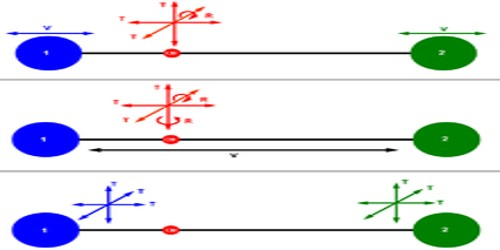# Degree of Freedom

Degree of freedom

Total number of independent or free motions that a body can have, these numbers are called degrees of freedom. It is an independent physical parameter in the formal description of the state of a physical system. The set of all dimensions of a system is known as a phase space, and degrees of freedom are sometimes referred to as its dimensions. For linear or translational motion degrees of freedom is 3. Rotational particles have 5 degrees of freedom.If from the total number of essential coordinates that is needed to express wholly the positions of the elements of a system the number of independent relation that exist among the elements is subtracted, then total degrees of freedom is available. For example, the number of molecules of a monoatomic independent system is n. Then degrees of freedom will be 3n, but the mutual positions of molecules are represented by nꞌ = (3n — r).

Molecular vibration, or the movement of atoms are defined by their degrees of freedom – which depends on the bonding states of said atoms. So every singular atom to complex molecules can move transnational along x,y,z called translational modes.